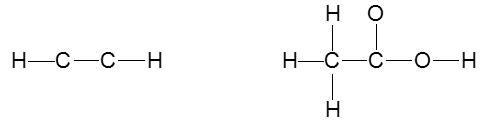Start typing, then use the up and down arrows to select an option from the list.# GOB Chemistry

Learn the toughest concepts covered in your GOB - General, Organic, and Biological Chemistry class with step-by-step video tutorials and practice problems.

Molecular Compounds

# Multiple Bonds (Simplified)

Single, double and/or triple bonds can be used in order to draw a correct Lewis Structure.

1

#### concept

Multiple Bonds (Simplified) Concept 11m
Play a video:
we're going to say in order to draw a correct lewis structure. Multiple bonds between elements are sometimes necessary. So here we have a single bond, a double bond and a triple bond. What we need to realize here first, in terms of their bond length, is notice that as we go from a single bond to a triple bond, the bond length is decreasing. Single bonds are the longest. Triple bonds are the shortest. In addition to this, realize that every time we make a co valent bond, it involves two electrons. So there's an electron here at this end and here at this end. So this tells us the number of valence electrons that are shared between the two atoms. Here in a single bond there are two valence electrons that are being shared between the two carbons. This equals one electron pair. In a double bottom we have two bonds. Each one again has two electrons. So that's a total of four valence electrons or two electron pairs. And then finally a triple bond has three bonds. Each one has two electrons. So that's a total of six valence electrons shared, which equates to three electron pairs.
2

#### example

Multiple Bonds (Simplified) Example 144s
Play a video: